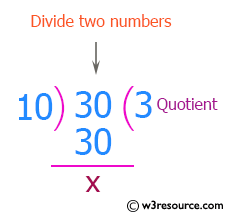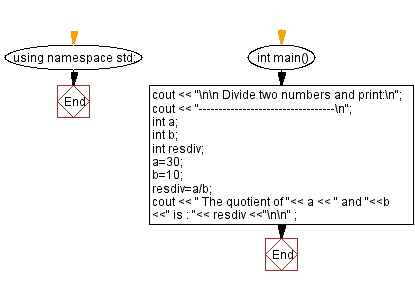﻿ C++ Exercises: Divide two numbers and print on the screen - w3resource

# C++ Exercises: Divide two numbers and print on the screen

## C++ Basic: Exercise-33 with Solution

Write a program in C++ to divide two numbers and print them on the screen.

Pictorial Presentation:Sample Solution:

C++ Code :

``````#include <iostream>
using namespace std;

int main()
{
cout << "\n\n Divide two numbers and print:\n";
cout << "----------------------------------\n";
int a;
int b;
int resdiv;
a=30;
b=10;
resdiv=a/b;
cout << " The quotient of "<< a << " and "<<b <<" is : "<< resdiv <<"\n\n" ;
}
``````

Sample Output:

```Divide two numbers and print:
----------------------------------
The quotient of 30 and 10 is : 3
```

Flowchart:C++ Code Editor:

What is the difficulty level of this exercise?

﻿

## C++ Programming: Tips of the Day

Why is there no std::stou?

The most pat answer would be that the C library has no corresponding "strtou", and the C++11 string functions are all just thinly veiled wrappers around the C library functions: The std::sto* functions mirror strto*, and the std::to_string functions use sprintf.

Ref: https://bit.ly/3wtz2qA

We are closing our Disqus commenting system for some maintenanace issues. You may write to us at reach[at]yahoo[dot]com or visit us at Facebook